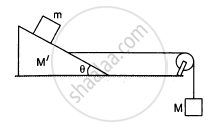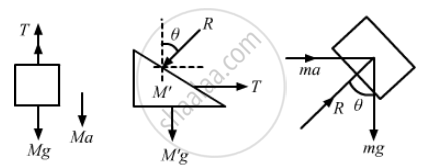# Find the Mass M of the Hanging Block in Figure (5−E16) that Will Prevent the Smaller Block from Slipping Over the Triangular Block. - Physics

Sum

Find the mass M of the hanging block in the following figure that will prevent the smaller block from slipping over the triangular block. All the surfaces are frictionless and the strings and the pulleys are light.#### Solution

The free-body diagram of the system is shown below:Block ‘m’ will have the same acceleration as that of M', as it does not slip over M'.

From the free body diagrams,
T + Ma – Mg = 0    ...(i)
T – M'a – Rsinθ = 0    ...(ii)

Rsinθ – ma = 0
Rcosθ – mg = 0
Eliminating T, R and a from the above equations, we get:
$M = \frac{M' + m}{\cot \theta - 1}$

Concept: Newton’s Second Law of Motion
Is there an error in this question or solution?

#### APPEARS IN

HC Verma Class 11, Class 12 Concepts of Physics Vol. 1
Chapter 5 Newton's Laws of Motion
Q 33 | Page 82